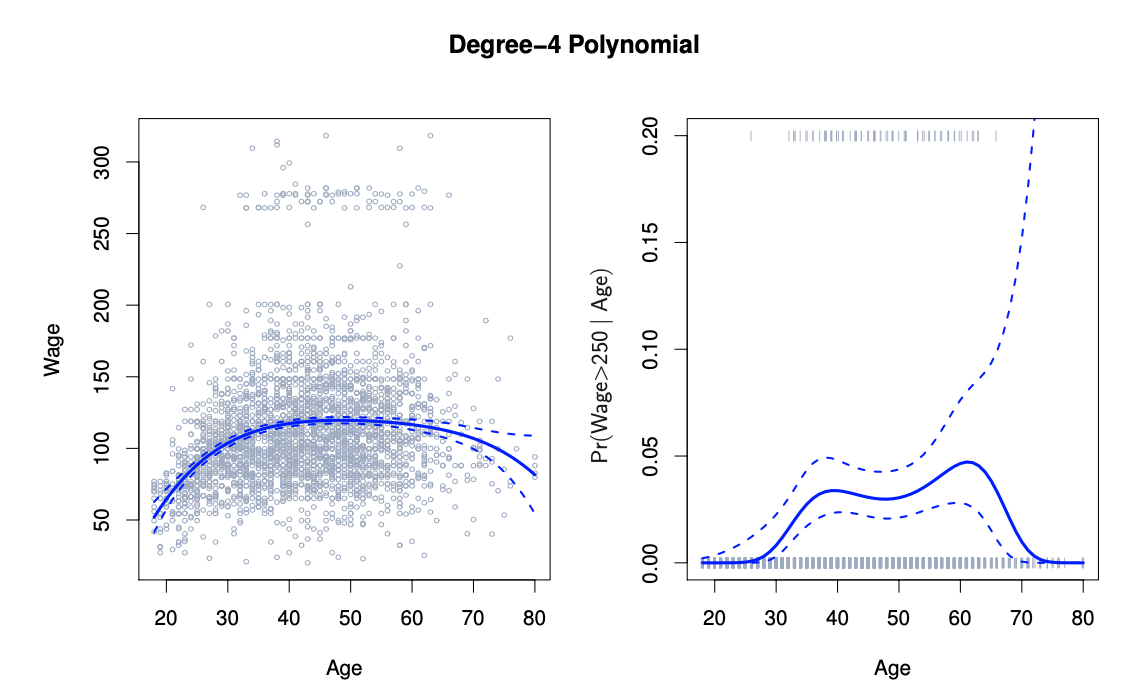## Moving Beyond Linearity

• The truth is almost never linear!

• Or almost never!
• But often the linearity assumption is “good enough”

• What about when its not?

• Polynomials

• Step Functions

• Splines

• Local Regression

• All of these models offer a lot of flexibility, without losing the ease and interpretability of linear models

## Polynominal Regression

• $$y_i = B_0 + B_1x_1 + B_2x_{i}^{2} + B_3x_{i}^{3} + ... + B_dx_{i}^{d} + \epsilon_i$$• Create new variables $$X_1 = X$$, $$X_2 = X^2$$, and so on, then treat as multiple linear regression

• Not really interested in the coefficients; more interested in the fitted function values at any value $$x_0$$:

• $$\hat{f}(x_0) = \hat{\beta_0} + \hat{\beta_1}x_0 + \hat{\beta_2}x_0^2 + \hat{\beta_3}x_3 + \hat{\beta_4}x_4$$
• Since $$\hat{f}(x_0)$$ is a linear function of the $$\hat{\beta_\ell}$$, can get a simple expression for pointwise-variances $$Var[\hat{f}(x_0)]$$ at any value of $$x_0$$. In the figure above, we have computed the fit and pointwise standard errors on a grid of values for $$x_0$$. We show $$\hat{f}(x_0) \pm 2 \cdot se[\hat{f}(x_0)]$$

• We either fix the degree $$d$$ at some reasonably low value, else use cross-validation to choose $$d$$

• Logistic regression follows naturally. For example, in the figure we model:

• $$Pr(y_i > 250|x_i) = \frac{exp(B_0 + B_1x_1 + B_2x_{i}^{2} + B_3x_{i}^{3} + ... + B_dx_{i}^{d})}{1 + exp(B_0 + B_1x_1 + B_2x_{i}^{2} + B_3x_{i}^{3} + ... + B_dx_{i}^{d})}$$

• To get confidence intervals, compute upper and lower bounds on on the logit scale, and then invert to get on probability scale

• Can do separately on several variables—just stack the variables into one matrix, and separate out the pieces afterwards (see GAMs later)

• Caveat: polynomials have notorious tail behavior — very bad for extrapolation

• Can fit using $$y ~ poly(x, degree = 3)$$ in formula

## Step Functions

• Another way of creating transformations of a variable — cut the variable into distinct regions

• $$C_1(X) = I(X < 35), C_2(X) = I(35 \leq X < 50), ... , C_3(X) = I(X \geq 65)$$